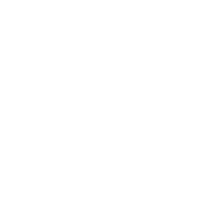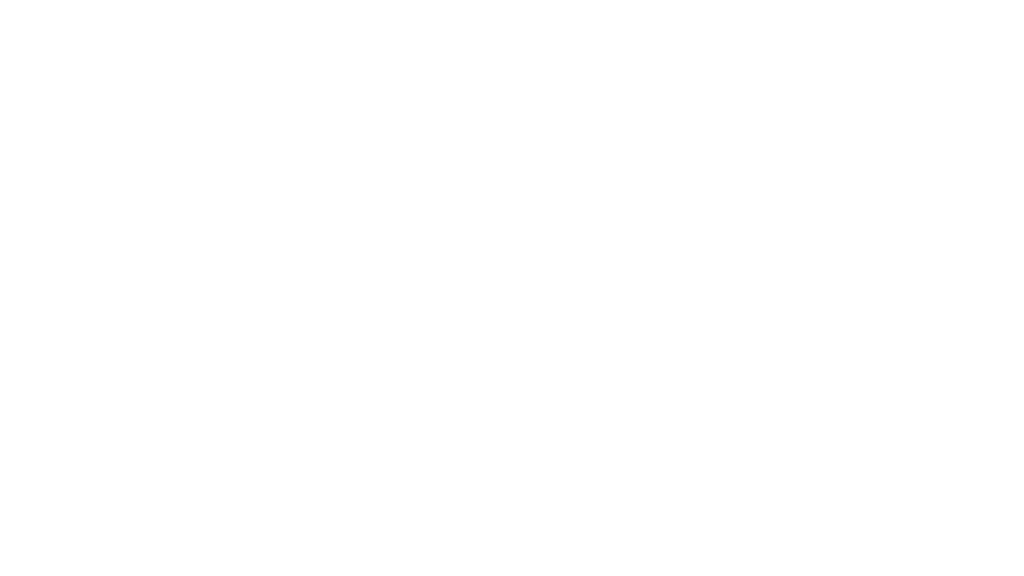# Real Numbers

##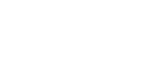1. Insert 4 rational numbers between 1 and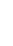without using formula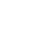Sol: we have to insert 4 rational numbers between 1 andand 1

4 +1 = 5

1 can be written as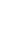Lcm of (4, 5) = 20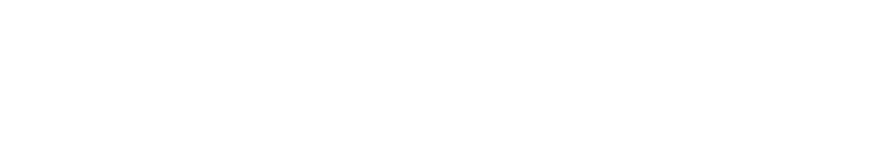2. The prime factorisation of a natural number (n) is 23 × 32 × 52 × 7. How many consecutive zeros will it have at the end of it? Justify your answer.

Sol:

n = 23 × 32 × 52 × 7

= 2 × 22 × 32 × 52 × 7

= 2 × 32 × 7 (52 × 22)

= 2 × 32 × 7 × (10)2 = 12600

∴ n has 2 consecutive zeros at the end

3. Find the value of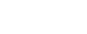Sol: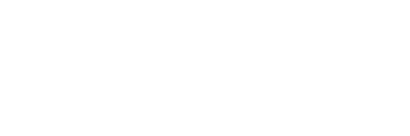4. Write any two irrational numbers between 3 and 4

Sol: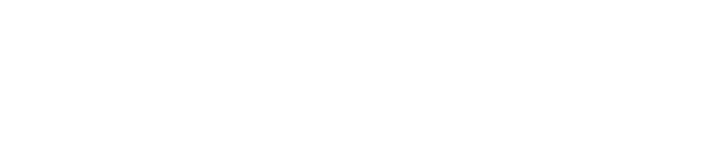5. Find the value of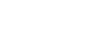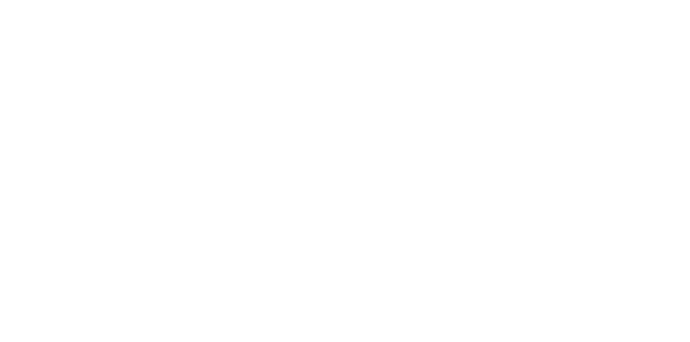6.  Find the HCF and LCM of 90, 144 by using the prime factorisation method

Sol: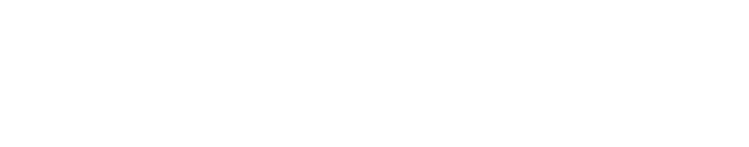7.  Is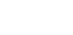rational or irrational?  justify your answer

Sol: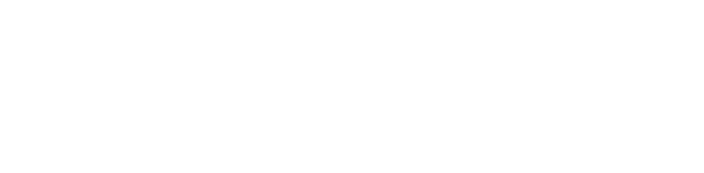8. Expand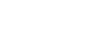Sol: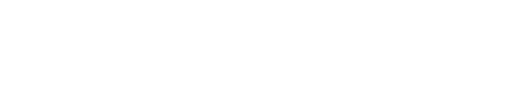9. Find the HCF of 24 and 33 by using division method

Sol:

33 > 24

33 = 24 × 1 + 9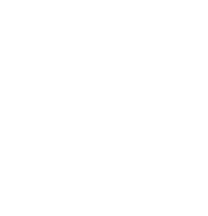24 = 9 × 2 + 6

9 = 6 × 1 + 3

6 = 3 × 2 + 0

∴ HCF of 24 and 33 = 3

10. Find the value of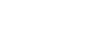Sol: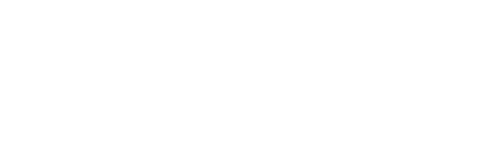11. Ramu says, “if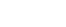= 0, the value of x is 0”. Do you agree with him? Give reason.

Sol: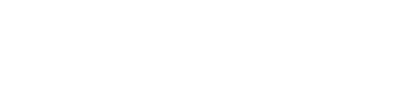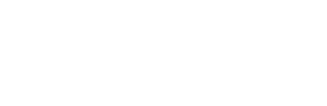####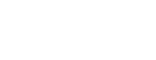1. Write any three numbers of two digits. Find the LCM and HCF for the above numbers by the prime factorisation method.

Sol: let 10, 12, 16 be three two-digit numbers

Prime factarisation of 10 = 2 × 5 = 21× 51

Prime factarisation of 12 = 2 × 2× 3 = 22 × 3

Prime factarisation of 16 = 2 × 2× 2× 2 = 24

HCF of 10, 12 and 16 = 21 = 2

LCM of 10, 12 and 16 = 24 × 3× 5 = 8 × 3× 5 = 120

2. Give an example for each of the following

(i) The product of two irrational numbers is a rational number

(ii) The product of two irrational numbers is an irrational number

Sol: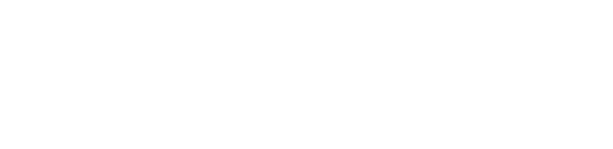#### 3. State with reasons which of the following are rational numbers and which are irrational numbers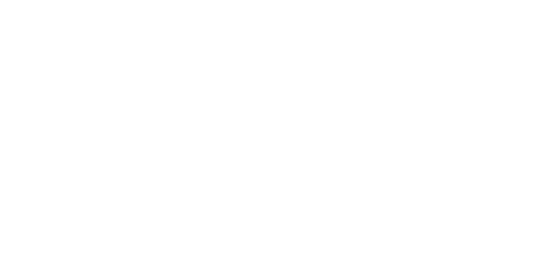#### 4. Expand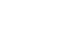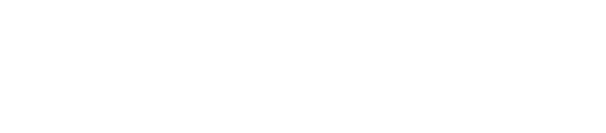#### Visit My Youtube Channel:  Click  on below  logo